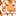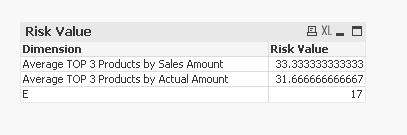Announcements
Sept. 27, 10AM ET Do more with Qlik, Insight Advisor – our intelligent AI-assistant in Qlik Sense: Register
cancel
Showing results for
Did you mean:Creator II

## How to Pass Condition values into ValueList()

Hi All,

How to achieve the below expression in a right way.

Expression:

Valuelist('Apple','Bannana','Carrot',if(wildmatch([fruits],'*G*'),[fruits]))

Thanks,

-Vidya

1 Solution

Accepted SolutionsMaster II

script change

dim

1

2

3

];

dimension

=pick(dim,'Average TOP 3 Products by Sales Amount','Average TOP 3 Products by Actual Amount',if(wildmatch(Product,'*E*'),Product))

expression

PICK(dim, Avg({<Product={"=rank(sum([Sales Amount]),Product)<=3"}>}[Risk Value]),Avg({<Product={"=rank(sum([Actual Amount]),Product)<=3"}>}[Risk Value]),SUM([Risk Value])
)

Learning never stops.
9 RepliesMaster IIIMaster II

where are you using this and what is the exact requirement of the below expression? there may be a easy way out.

if you are using it in the dimension try this

in the script

dim

1

2

3

4

];

in the dimension

pick(dim,'Apple','Bannana','Carrot',if(wildmatch([fruits],'*G*'),[fruits]))

Learning never stops.Creator II
Author

With the expression, i was able to achieve the below result set.

Table:

 Product Sales Amount Actual Amount Risk Value Return Value A 60 5 20 15 B 50 100 25 25 C 70 70 55 35 D 20 35 15 30 E 10 25 17 15 F 5 30 20 5

Dimension:

=ValueList('AUM','Flow')

Measure:

if(ValueList('AUM','Flow') =

'AUM', Avg({<Product={"=rank(sum([Sales Amount]),Product)<=3"}>}[Risk Value]),

if(ValueList('AUM','Flow') =

'Flow',Avg({<Product={"=rank(sum([Actual Amount]),Product)<=3"}>}[Risk Value])

))

Result:

 Dimension Risk Vlaue Average TOP 3 Products by Sales Amount 33.33 Average TOP 3 Products by Actual Amount 31.66

Here is it trick:

Now I want to show my own product in the result table.

Example:

 Dimension Risk Vlaue Average TOP 3 Products by Sales Amount 33.33 Average TOP 3 Products by Actual Amount 31.66 E 17

Thanks,

-VidyaLuminary Alumni

Hi,

ValueList() only accepts discrete values, so you cannot use If inside Valuelist(). Try fixing this on data model level by creating a separate table linked to your data which will contain those "fruits" you need and will connect to respective values in your data model.

Hope this helps.

JurajMaster II

Is this what you are looking for?Learning never stops.Master II

script change

dim

1

2

3

];

dimension

=pick(dim,'Average TOP 3 Products by Sales Amount','Average TOP 3 Products by Actual Amount',if(wildmatch(Product,'*E*'),Product))

expression

PICK(dim, Avg({<Product={"=rank(sum([Sales Amount]),Product)<=3"}>}[Risk Value]),Avg({<Product={"=rank(sum([Actual Amount]),Product)<=3"}>}[Risk Value]),SUM([Risk Value])
)

Learning never stops.Creator II
Author

Hi All,

I have found the solution. Thanks to everyone and special thanks to Juraj for giving me this idea.

Step1: Created Inline Table with column names DimID, DIM values

Step2: Created Resident with only the products which I was interested in.

for example:

[Performance Statistics]:

load if(WildMatch("Furits",'*E*'),"Product ID") as Dim,if(WildMatch("Fruits",'*E*'),"Product Name") as DimValue Resident [Fruits_Table];

Step3: Concatenated Resident with Inline table.

Now that I have my table ready. I used the following expression to achieve my requirement.

Dimensions:

=if([Product ID]=Dim,DimValue,

if(Dim=1,DimValue,

if(Dim=2,DimValue,

if(Dim=3,DimValue

))))

Measures:

if(Dim=1 , Avg({<[Product ID]={"=rank(sum([Sales Amount]),[Product ID])<=10"}>}[Risk Value]),

if(Dim=2 , Avg({<[Product ID]={"=rank(sum([Actual Amount]),[Product ID])<=10"}>}[Risk Value]),

if(Dim=3 , Avg({<[Product ID]={"=rank(-sum([Actual Amount]),[Product ID])<=10"}>}[Risk Value]),

([Risk Value])

)

))

Result:

 Dimension Risk Value Average TOP 3 Products by Sales Amount 33.33 Average TOP 3 Products by Actual Amount 31.66 E 17

Thanks,

-VidyaMaster II

Your way is one way of achieving it my friend. But if you use pick() with no nested if it will be optimized and faster as well.

Good that you found a solution.Learning never stops.Creator II
Author